## 7.11Enhancements to the k-omega model

A comparison of the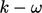and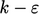models shows the dissipation term is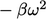in Eq. (7.37 ) for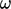and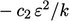in Eq. (7.2 ) for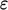. While theterm behaves well, theterm is troublesome at a wall as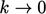, so requires damping in the low-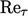formulation in Sec. 7.9 to resolve the viscous sub-layer.

Its better dissipation term gives the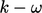model an advantage over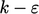in the near-wall region. The disadvantage the originalmodel is its sensitivity to the freestream values of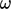, which is not present in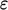in themodel. Neither model, in their original form, performs well under adverse pressure gradients.

However, since its initial publication, many enhancements have been made to the original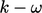model, in particular to address the problems mentioned above.

### Cross-diﬀusion

The dependency on freestream values ofis addressed by the inclusion of a cross-diﬀusion term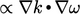in theequation.17 The term is derived when the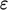equation, e.g. Eq. (7.2 ), is expressed in terms of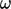by substituting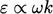. Its form is due to the expansion of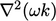in the diﬀusion term by Eq. (2.74b ).

The cross-diﬀusion term makes theequation more equivalent to, and thus independent of freestream values.

### Stress limiter

The original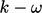and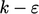models are known to delay or suppress ﬂow separation under adverse pressure gradients (described in Sec. 6.5 ). Under such conditions the ratio of the production to dissipation of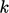can be signiﬁcantly higher than unity. The calculated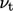from Eq. (7.39 ) is excessively high, causing an over-prediction of shear stress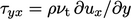.

The problem is alleviated by limiting the shear stress, based on the assumption it is proportional to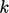in the boundary layer, i.e.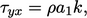where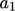is a constant. A stress limiter is implemented through a modiﬁcation to the calculation of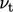: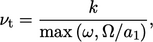(7.43)
where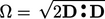is a 3D representation of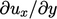.

### Standard models

Diﬀerent versions of the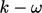model are well catalogued in the Turbulence modeling resource, NASA Langley Research Center, https://turbmodels.larc.nasa.gov.

Today, there are arguably two “standard”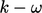models, First, the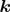-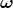SST model,18 (SST = shear stress transport) which emerged as a popular choice in CFD over recent decades.

It combines the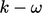model near the wall with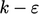(expressed in terms of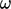) in the freestream, by applying blending functions to model coeﬃcients, the cross-diﬀusion term and the stress limiter.

Secondly, the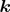-2006 model19 applies the cross-diﬀusion term and stress limiting to the originalmodel. The terms are applied using switches so that the model maintains its simplicity, without the need for blending functions.

17Florian Menter, Zonal two equation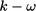models for aerodynamic ﬂows, 1993.
18Florian Menter, Two-equation eddy-viscosity turbulence models for engineering applications, 1994.
19David Wilcox, Turbulence modeling for CFD, 3rd ed., 2006.

Notes on CFD: General Principles - 7.11 Enhancements to the k-omega model